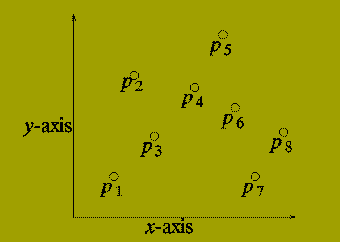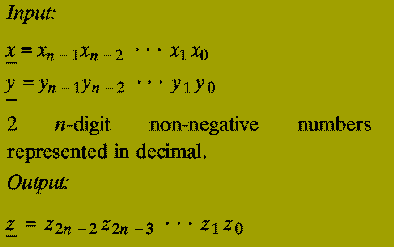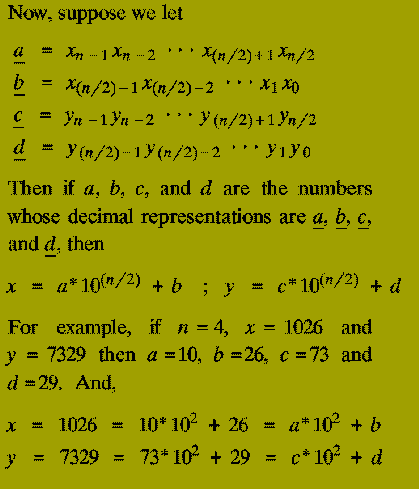### Divide-and-Conquer

This is a method of designing algorithms that (informally) proceeds as follows:

Given an instance of the problem to be solved, split this into several, smaller, sub-instances (of the same problem) independently solve each of the sub-instances and then combine the sub-instance solutions so as to yield a solution for the original instance. This description raises the question:

By what methods are the sub-instances to be independently solved?

The answer to this question is central to the concept of Divide-&-Conquer algorithm and is a key factor in gauging their efficiency.

Consider the following: We have an algorithm, alpha say, which is known to solve all problem instances of size n in at most c n^2 steps (where c is some constant). We then discover an algorithm, beta say, which solves the same problem by:

• Dividing an instance into 3 sub-instances of size n/2.
• Solves these 3 sub-instances.
• Combines the three sub-solutions taking d n steps to do this.
Suppose our original algorithm, alpha, is used to carry out the `solves these sub-instances' step 2. Let

T(alpha)( n ) = Running time of alpha

T(beta)( n ) = Running time of beta

Then,

T(alpha)( n ) = c n^2 (by definition of alpha)

But

```
T(beta)( n )  = 3 T(alpha)( n/2 ) + d n

= (3/4)(cn^2) + dn
```

So if dn < (cn^2)/4 (i.e. d < cn/4) then beta is faster than alpha

In particular for all large enough n, (n > 4d/c = Constant), beta is faster than alpha.

This realisation of beta improves upon alpha by just a constant factor. But if the problem size, n, is large enough then

```
n     >   4d/c
n/2   >   4d/c
...
n/2^i >   4d/c

```

which suggests that using beta instead of alpha for the `solves these' stage repeatedly until the sub-sub-sub..sub-instances are of size n0 < = (4d/c) will yield a still faster algorithm.

So consider the following new algorithm for instances of size n

```procedure gamma (n : problem size ) is
begin
if n <= n^-0 then
Solve problem using Algorithm alpha;
else
Split into 3 sub-instances of size n/2;
Use gamma to solve each sub-instance;
Combine the 3 sub-solutions;
end if;
end gamma;
```

Let T(gamma)(n) denote the running time of this algorithm.

```
cn^2                  if n < = n0
T(gamma)(n)  =
3T(gamma)( n/2 )+dn   otherwise

```

We shall show how relations of this form can be estimated later in the course. With these methods it can be shown that

T(gamma)( n ) = O( n^{log3} ) (=O(n^{1.59..})

This is an asymptotic improvement upon algorithms alpha and beta.

The improvement that results from applying algorithm gamma is due to the fact that it maximises the savings achieved beta.

The (relatively) inefficient method alpha is applied only to "small" problem sizes.

The precise form of a divide-and-conquer algorithm is characterised by:

• The threshold input size, n0, below which the problem size is not sub-divided.
• The size of sub-instances into which an instance is split.
• The number of such sub-instances.
• The algorithm used to combine sub-solutions.

In (II) it is more usual to consider the ratio of initial problem size to sub-instance size. In our example this was 2. The threshold in (I) is sometimes called the (recursive) base value. In summary, the generic form of a divide-and-conquer algorithm is:

```procedure D-and-C (n : input size) is
begin
if n < = n0 then
Solve problem without further
sub-division;
else
Split into r sub-instances
each of size n/k;
for each of the r sub-instances do
D-and-C (n/k);
Combine the r resulting
sub-solutions to produce
the solution to the original problem;
end if;
end D-and-C;
```

Such algorithms are naturally and easily realised as:

### Recursive Procedures

in (suitable) high-level programming languages.

## Example 1:

### Binary Search

Consider the following problem: one has a directory containing a set of names and a telephone number associated with each name.

The directory is sorted by alphabetical order of names. It contains n entries which are stored in 2 arrays:

names (1..n) ; numbers (1..n)

Given a name and the value n the problem is to find the number associated with the name.

We assume that any given input name actually does occur in the directory, in order to make the exposition easier.

The Divide-&-Conquer algorithm to solve this problem is the simplest example of the paradigm.

It is based on the following observation

Given a name, X say,

X occurs in the middle place of the names array

Or

X occurs in the first half of the names array. (U)

Or

X occurs in the second half of the names array. (L)

U (respectively L) are true only if X comes before (respectively after) that name stored in the middle place.

This observation leads to the following algorithm:

```function search (X : name;
start, finish : integer)
return integer is
middle : integer;
begin
middle := (start+finish)/2;
if names(middle)=x then
return numbers(middle);
elsif X<names(middle) then
return search(X,start,middle-1);
else -- X>names(middle)
return search(X,middle+1,finish);
end if;
end search;
```

Exercise: How should this algorithm be modified to cater for the possibility that a given name does not occur in the directory? In terms of the generic form of divide-&-conquer algorithms, at which stage is this modification made?

We defer analysis of the algorithm's performance until later.

## Example 2

### Closest Pair

Input:

```
P  =  {p(1), p(2) ,..., p(n) }
```

where p(i) = ( x(i), y(i) ).

A set of n points in the plane.

Output

The distance between the two points that are closest.

Note: The distance DELTA( i, j ) between p(i) and p(j) is defined by the expression:

```Square root of { (x(i)-x(j))^2 + (y(i)-y(j))^2 }
```

We describe a divide-and-conquer algorithm for this problem.We assume that:

n is an exact power of 2, n = 2^k.

For each i, x(i) < = x(i+1), i.e. the points are ordered by increasing x from left to right.

Consider drawing a vertical line (L) through the set of points P so that half of the points in P lie to the left of L and half lie to the right of L.There are three possibilities:

• The closest pair lie in P-LEFT.
• The closest pair lie in P-RIGHT.
• The closest pair contains:

One Point from P-LEFT

and

One Point from P-RIGHT

So we have a (rough) Divide-and-Conquer Method as follows:

```function closest_pair (P: point set; n: in
teger )
return float is
DELTA-LEFT, DELTA-RIGHT : float;
DELTA : float;
begin
if n = 2 then
return distance from p(1) to p(2);
else
P-LEFT  := ( p(1), p(2) ,..., p(n/2) );
P-RIGHT := ( p(n/2+1), p(n/2+2) ,..., p(n) );
DELTA-LEFT := closestpair( P-LEFT, n/2 );
DELTA-RIGHT := closestpair( P-RIGHT, n/2 );
DELTA := minimum ( DELTA-LEFT, DELTA-RIGHT );
--*********************************************
Determine whether there are points p(l) in
P-LEFT and p(r) in P-RIGHT with
distance( p(l), p(r) ) < DELTA. If there
are such points, set DELTA to be the smallest
distance.
--**********************************************
return DELTA;
end if;
end closest_pair;

```

The section between the two comment lines is the `combine' stage of the Divide-and-Conquer algorithm.

If there are points p(l) and p(r) whose distance apart is less than DELTA then it must be the case that

• The x-coordinates of p(l) and p(r) differ by at most DELTA.
• The y-coordinates of p(l) and p(r) differ by at most DELTA.The combine stage can be implemented by:

• Finding all points in P-LEFT whose x-coordinate is at least x(n/2)-DELTA.
• Finding all points in P-RIGHT whose x-coordinate is at most x(n/2)+DELTA.

Call the set of points found in (1) and (2) P-strip. and sort the s points in this in order of increasing y-coordinate. letting ( q(1),q(2) ,..., q(s) ) denote the sorted set of points.

Then the combine stage of the algorithm consists of two nested for loops:

```for i in 1..s loop
for j in i+1..s loop
exit when (| x(i) - x(j) | > DELTA or
| y(i) - y(j) | > DELTA);
if distance( q(i), q(j) ) < DELTA then
DELTA := distance ( q(i), q(j) );
end if;
end loop;
end loop;
```

## Example 3

### Integer Multiplication

Divide-and-Conquer may also be applied to problems other than those involving searching. The following problem should be familiar:The (2n)-digit decimal representation of the product x * y.

Note: The algorithm below works for any number base, e.g. binary, decimal, hexadecimal, etc. We use decimal simply for convenience.

The classical primary school algorithm for multiplication requires O( n^2 ) steps to multiply two n-digit numbers.

A step is regarded as a single operation involving two single digit numbers, e.g. 5+6, 3*4, etc.

In 1962, A.A. Karatsuba discovered an asymptotically faster algorithm for multiplying two numbers by using a divide-and-conquer approach.From this we also know that the result of multiplying x and y (i.e. z) isThe terms ( a*c ), ( a*d ), ( b*c ), and ( b*d ) are each products of

### 2 (n/2)-digit numbers.

Thus the expression for the multiplication of x and y in terms of the numbers a, b, c, and d tells us that:

• Two single digit numbers can be multiplied immediately. (Recursive base: 1 step)
• If n > 1 then the product of 2 n-digit numbers can be expressed in terms of 4 products of 2 (n/2)-digit numbers (Divide-and-Conquer stage)
• To calculate the result of multiplying x and y given the four products returned involves only addition (can be done in O( n ) steps) and multiplying by a power of 10 (also can be done in O( n ) steps, since it only requires placing the appropriate number of 0s at the end of the number). (Combine stage).

(1-3) therefore describe a Divide-&-Conquer algorithm for multiplying two n-digit numbers represented in decimal. However,

Moderately difficult: How many steps does the resulting algorithm take to multiply two n-digit numbers?

Karatsuba discovered how the product of 2 n-digit numbers could be expressed in terms of three products each of 2 (n/2)-digit numbers - instead of the four products that a naive implementation of the Divide-and-Conquer schema above uses.

This saving is accomplished at the expense of slightly increasing the number of steps taken in the `combine stage' (Step 3) (although, this will still only use O( n ) operations).

Suppose we compute the following 3 products (of 2 (n/2)-digit numbers):```function Karatsuba  (xunder, yunder : n-digit integer;
n : integer)
return (2n)-digit integer is
a, b, c, d : (n/2)-digit integer
U, V, W : n-digit integer;
begin
if n = 1 then
return x(0)*y(0);
else
a := x(n-1) ... x(n/2);
b := x(n/2-1) ... x(0);
c := y(n-1) ... y(n/2);
d := y(n/2-1) ... y(0);
U := Karatsuba ( a, c, n/2 );
V := Karatsuba ( b, d, n/2 );
W := Karatsuba ( a+b, c+d, n/2 );
return U*10^n + (W-U-V)*10^n/2 + V;
end if;
end Karatsuba;
```

### Performance Analysis

It was observed earlier that one reason for examining algorithmic paradigms was the fact that their running time could often be precisely determined.

This is useful in allowing comparisons between the performances of two algorithms to be made.

For Divide-and-Conquer algorithms the running time is mainly affected by 3 criteria:

• The number of sub-instances (alpha) into which a problem is split.
• The ratio of initial problem size to sub-problem size. (beta)
• The number of steps required to divide the initial instance and to combine sub-solutions, expressed as a function of the input size, n.

Suppose, P, is a divide-and-conquer algorithm that instantiates alpha sub-instances, each of size n/beta.

Let Tp( n ) denote the number of steps taken by P on instances of size n. Then

```
Tp( n0 )  =  Constant   (Recursive-base)
Tp( n )   =  alpha Tp( n/beta ) + gamma( n )

```

In the case when alpha and beta are both constant (as in all the examples we have given) there is a general method that can be used to solve such recurrence relations in order to obtain an asymptotic bound for the running time Tp( n ).

In general:

```
T( n )  =  alpha T( n/beta ) + O( n^gamma )
```

(where gamma is constant) has the solution

```
O(n^gamma)             if   alpha < beta^gamma
T( n )  =   O(n^gamma log n)       if   alpha = beta^gamma}
O(n^{log^-beta(alpha)) if   alpha > beta^gamma

```PED Home Page